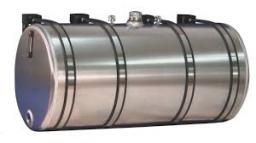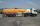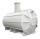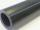# Tank diameter

A cylindrical tank has a volume of 60 hectoliters and is 2.5 meters deep. Calculate the tank diameter.

D =  1.7481 m

### Step-by-step explanation:Did you find an error or inaccuracy? Feel free to write us. Thank you!Tips to related online calculators
Do you know the volume and unit volume, and want to convert volume units?

## Related math problems and questions:

• Cylindrical tankCylindrical tank holds 600hl water and is deep 2.5 m. Calculate the diameter of the cylinder.
• Gasoline tank cylindricalWhat is the inner diameter of the tank, which is 8 m long and contains 40 cubic cubic meters of gasoline?
• HectolitersHow deep is the pool if there are 2025 hectoliters of water and the bottom dimensions are a = 15 meters b = 7.5 meters and the water level is up to 9/10 (nine-tenths) of height?
• Hectoliters of waterThere are 942 hectoliters of water in a cylindrical tank with an inner diameter of 6 m. The water reaches two thirds of the depth of the tank. Calculate its depth.
• Cylinder heightCalculate the height of the cylinder and its surface is 2500 dm2 and the bases have a diameter 5dm.
• Cylinder:Cylinder: calculate the height of a 3 liter cylindrical pot with a diameter of 10 cm.
• CylinderIn a 1-meter diameter cylinder is 1413 liters of water, which is 60% of the cylinder. Calculate the cylinder height in meters, do not write the units. The resulting value round and write as an integer.
• Diameter = heightThe cylinder's surface, the height of which is equal to the diameter of the base, is 4239 cm square. Calculate the cylinder volume.
• Water tank 2Water tank cuboid is 12 meters long and 6.5 meters wide and 1.2 meters high. How many hectoliters are in the tank when it is filled to 81%?
• The pipeThe pipe is 1.5 m long. Its outer diameter is 60 cm, inner diameter is 52 cm. Calculate the pipe's weight if the material's density from which it is made is 2 g/cm3. Round the results to whole kilograms.
• Horizontal Cylindrical SegmentHow much fuel is in the horizontal cylindrical segment tank with a length of 10m, the width of level 1 meter, and the level is 0.2 meters below the tank's upper side?
• Tank and waterWe poured a cylindrical tank with 3.5 liters of water. If the tank base diameter is 3 dm, how height is the water level in?
• Cylindrical tank9.6 hl of water is poured into a cylindrical tank with a bottom diameter of 1.2 m. What height in centimeters does the water reach?
• Water tankThe water tank has a cylindrical shape with a base diameter of 4.2 m and is 80 cm deep. How many minutes will take fill it 10 cm below the edge of the tank if water flowing 2 liters per second?
• Equilateral cylinderEquilateral cylinder (height = base diameter; h = 2r) has a volume of V = 199 cm3 . Calculate the surface area of the cylinder.
• Height as diameter of baseThe rotary cylinder has a height equal to the base diameter and a surface of 471 cm2. Calculate the volume of a cylinder.
• CylinderThe cylinder surface is 922 dm2. Its height is equal to the radius of the base. Calculate the height of this cylinder.